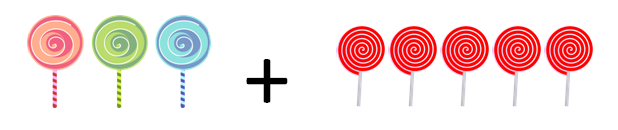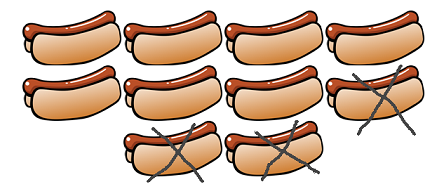# Math Word Problems: Lesson for Kids

Instructor: Audrey Akins

Audrey has more than a decade of experience teaching elementary. She has a bachelor's in journalism and a master's in education.

Once you've learned to add, subtract, multiply, and divide, the next step is learning how to apply those skills when solving word problems. This lesson will teach you how to recognize some of the different types of word problems.

## Understanding Word Problems

When solving any type of word problem, pay attention to the action in the problem. This will tell you whether or not you are joining things together, taking things apart, determining the total amount of things, or deciding how many of an item each person should get.

## Addition and Subtraction Word Problems

In some word problems, you'll know you're supposed to add because the action will indicate that two things are being joined together. For example, let's pretend it's your birthday. You get 3 lollipops from your mom, and then your grandma gives you 5 more lollipops. If you want to know how many you have in all, you add because you have to join the two groups of lollipops together to determine the total amount: 3 + 5 = 8. Some addition word problems also end with questions like: 1) How many in all? or 2) How many altogether?Now let's look at what happens when you're subtracting. When you subtract, the action you need to pay attention to is taking things away from the group. Since it's your birthday, you've decided to have a party. Before the party starts, your mom makes 10 hot dogs. During the party, your friends eat 7 hot dogs. In order to solve this problem, you'd subtract because you're taking 7 hot dogs away from the group of 10 that you started with: 10 - 7 = 3.Another way to determine if you'll have to subtract is if there is a missing part. Let's say you begin the party with 18 balloons and end the party with 6. The number of balloons that are gone is the missing part. If you have to subtract, that can tell you how many balloons your friends took home: 18 - 6 = 12, so 12 balloons are gone.

To unlock this lesson you must be a Study.com Member.

### Register to view this lesson

Are you a student or a teacher?